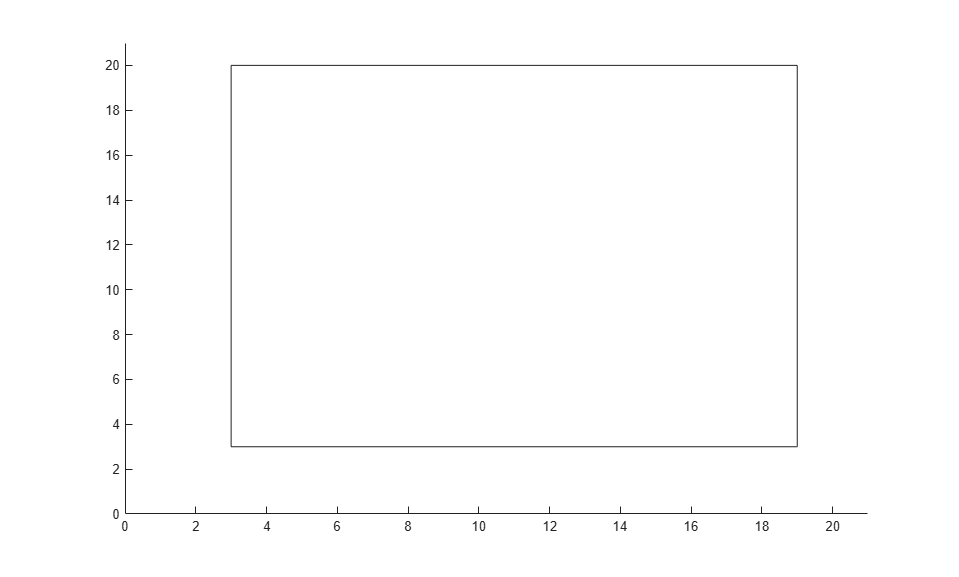# Custom Output Function for Genetic Algorithm

This example shows the use of a custom output function in the genetic algorithm solver `ga`. The custom output function performs the following tasks:

• Plot the range of the first two components of the population as a rectangle. The left and lower sides of the rectangle are at the minima of `x(1)` and `x(2)` respectively, and the right and upper sides are at the respective maxima.

• Halt the iterations when the best function value drops below `0.1` (the minimum value of the objective function is `0`).

• Record the entire population in a variable named `gapopulationhistory` in your MATLAB® workspace every 10 generations.

• Modify the initial crossover fraction to the custom value `0.2`, and then update it back to the default `0.8` after 25 generations. The initial setting of `0.2` causes the first several iterations to search primarily at random via mutation. The later setting of `0.8` causes the following iterations to search primarily via combinations of existing population members.

### Objective Function

The objective function is for four-dimensional `x` whose first two components are integer-valued.

```function f = gaintobj(x) f = rastriginsfcn([x(1)-6 x(2)-13]); f = f + rastriginsfcn([x(3)-3*pi x(4)-5*pi]); ```

### Output Function

The custom output function sets up the plot during initialization, and maintains the plot during iterations. The output function also pauses the iterations for `0.1s` so you can see the plot as it develops.

```function [state,options,optchanged] = gaoutfun(options,state,flag) persistent h1 history r optchanged = false; switch flag case 'init' h1 = figure; ax = gca; ax.XLim = [0 21]; ax.YLim = [0 21]; l1 = min(state.Population(:,1)); m1 = max(state.Population(:,1)); l2 = min(state.Population(:,2)); m2 = max(state.Population(:,2)); r = rectangle(ax,'Position',[l1 l2 m1-l1 m2-l2]); history(:,:,1) = state.Population; assignin('base','gapopulationhistory',history); case 'iter' % Update the history every 10 generations. if rem(state.Generation,10) == 0 ss = size(history,3); history(:,:,ss+1) = state.Population; assignin('base','gapopulationhistory',history); end % Find the best objective function, and stop if it is low. ibest = state.Best(end); ibest = find(state.Score == ibest,1,'last'); bestx = state.Population(ibest,:); bestf = gaintobj(bestx); if bestf <= 0.1 state.StopFlag = 'y'; disp('Got below 0.1') end % Update the plot. figure(h1) l1 = min(state.Population(:,1)); m1 = max(state.Population(:,1)); l2 = min(state.Population(:,2)); m2 = max(state.Population(:,2)); r.Position = [l1 l2 m1-l1 m2-l2]; pause(0.1) % Update the fraction of mutation and crossover after 25 generations. if state.Generation == 25 options.CrossoverFraction = 0.8; optchanged = true; end case 'done' % Include the final population in the history. ss = size(history,3); history(:,:,ss+1) = state.Population; assignin('base','gapopulationhistory',history); end ```

### Problem Setup and Solution

Set the lower and upper bounds.

```lb = [1 1 -30 -30]; ub = [20 20 70 70]; ```

Set the integer variables and number of variables.

```intcon = [1 2]; nvar = 4; ```

Set options to call the custom output function, and to initially have little crossover.

```options = optimoptions('ga','OutputFcn',@gaoutfun,'CrossoverFraction',0.2); ```

For reproducibility, set the random number generator.

```rng default ```

Set the objective function and call the solver.

```fun = @gaintobj; [x,fval] = ga(fun,nvar,[],[],[],[],lb,ub,[],intcon,options) ```
```Got below 0.1 Optimization terminated: y x = 6.0000 13.0000 9.4201 15.7052 fval = 0.0059 ```The output function halted the solver.

View the size of the recorded history.

```disp(size(gapopulationhistory)) ```
``` 40 4 6 ```

There are six records of the 40-by-4 population matrix (40 individuals, each a 4-element row vector).

## SupportGet trial now